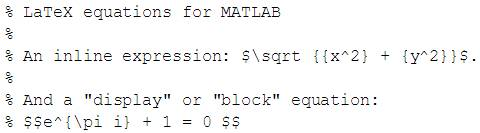# Transfer equations from MathType to Matlab

• MATLAB
Hi,

Has anyone tried to paste equations from MathType into Matlab ? I follow every step described here

With MathType and MATLAB you can:
• Add an equation to a MATLAB function file: Copy-and-paste equations and expressions from MathType directly into a MATLAB function file as TeX. How-to
• Copy equations from MATLAB function files: Copy-and-paste an equation from a MATLAB function file into MathType and it should be ready to edit or use in a new document. How-to
Step-by-step instructions:
Setting up MathType to work with MATLAB
1. From MathType's Preferences menu choose: Cut and Copy Preferences. On the Macintosh, this will be in the Preferences flyout of the MathType menu.
2. In the MathML or TeX group, choose: LaTeX 2.09 and later. Click OK.
3. Choose Format > Inline Equation. Note that MATLAB versions R2010b and earlier are only capable of display (or block) equations, but the steps below will be easier if you'll set MathType's Inline Equation property regardless.
Add an equation to a MATLAB function file
1. To annotate a function file with an equation, begin the line with a % symbol.
2. After creating the equation in MathType, select and copy it.
3. Paste it into the function file. For example:4. You'll need to manually insert an extra \$ before and after a block equation, as you see in the example.
Depending on which MATLAB "contributed files" you may have installed, there may be additional capability for MathType equations, such as MathML.

No matter what equation I paste, I get following error in Matlab:

Code:
|
Error: The input character is not valid in MATLAB statements or expressions.

## Answers and Replies

kreil
Gold Member
Are you pasting into a function file? That looks like an error you would get in the command window.

Are you pasting into a function file? That looks like an error you would get in the command window.
No I am not. I thought Matlab deals with the concept the same. Was I wrong?
An equation definition in command window should be as valid as the same equation annotation in *.m file. (perhaps)

kreil
Gold Member
Yes they are subtly different in a few ways. Function files (.m files) are reusable, so you can write a function and save it and call it whenever you want. You can't do that with functions you define at the command line (well you can make an anonymous function, but it only lasts as long as you have the variable around...). You can add comments to your code, debug, and do other stuff with function files that you can't do in the command window as well.

Since these equations you want to write are really comments in a file, entered as LaTeX code, entering them in the command window doesn't really work.

Since these equations you want to write are really comments in a file, entered as LaTeX code, entering them in the command window doesn't really work.

Well, that's the point. As you know adding a % character in the beginning of line in MATLAB, will make that entire line as a comment and will be ignored for interpretation purpose. How above sample code is suppose to define me a function where the function definition line is already commented out? That's my question I am afraid.

kreil
Gold Member
When you publish the file it turns the comments into HTML, so the equation renders and displays. The m file is just the source file that is published to produce the final output

hmmm. I see.

Thanks for all the replies Kreil.

kreil
Gold Member
Just to be clear, you can't import an equation into MATLAB as a usable function from math type. You'll need to do that manually by writing the expression out (or use an anonymous function). The functionality described above allows you to display the same equation as an annotation.

Please let me know if that answers your question